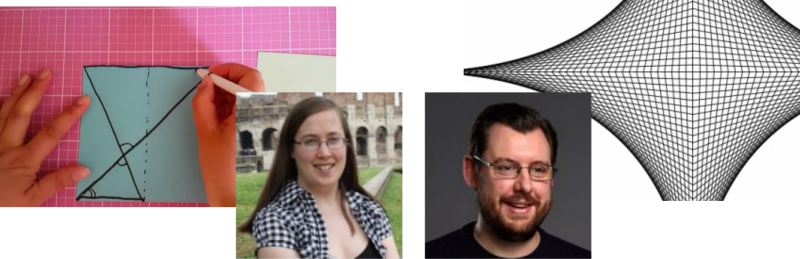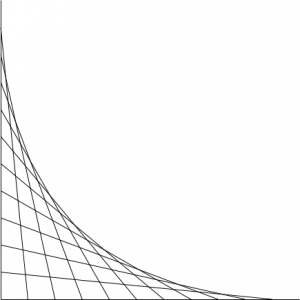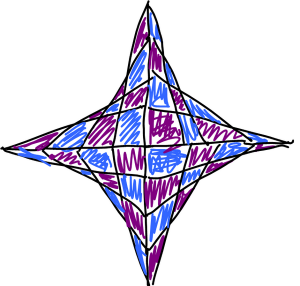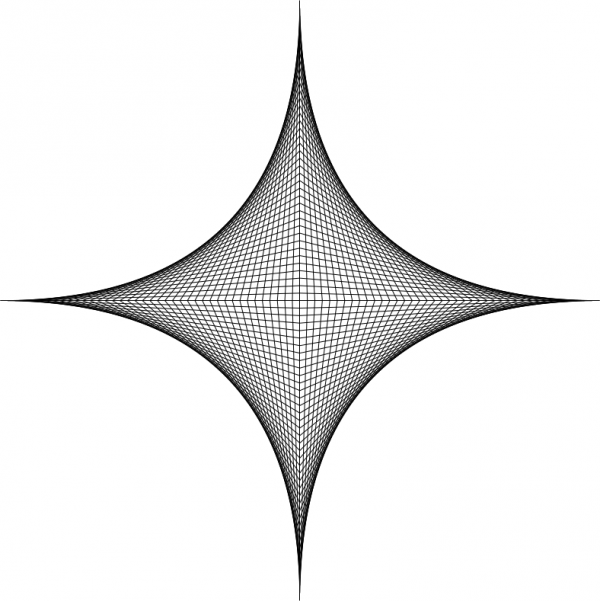# The Big Internet Math-Off, Round 1 – Peter Rowlett v Alison KiddleHere’s the third match in Round 1 of The Big Internet Math-Off. Today, we’re pitting Peter Rowlett against Alison Kiddle.

Take a look at both pitches, vote for the bit of maths that made you do the loudest “Aha!”, and if you know any more cool facts about either of the topics presented here, please write a comment below!

### Peter Rowlett – Parabolic curvesPeter Rowlett is a lecturer at Sheffield Hallam University, co-host of the dearly missed Math/Maths podcast, and one of the three people who keeps this site running. You can find him on Twitter at @peterrowlett, or at peterrowlett.net.

When I was nine, a few of us at school were sent to a taster session with the teacher into whose class we would be moving in a few months. I didn’t actually go into that class, because we moved house and I moved schools, but I do remember what the taster involved.

We sat around a table with the teacher. He asked us to draw axes (let’s call them $x$ and $y$) on graph paper, labelling points $0$–$10$ on each axis. We were instructed to draw a straight line with a ruler from $(0,10)$ to $(1,0)$. Then from $(0,9)$ to $(2,0)$, then $(0,8)$ to $(3,0)$, and so on. I suppose this is $(0,11-c)$ to $(c,0)$ for integers $0 < c \le 10$.

The result is a nice figure, and he made the point that through the whole process we had only drawn straight lines, yet what we had drawn was clearly a curve. Astonishing!If you extend this concept into the other three quadrants, you get a nice figure, and you can colour lines or chessboard-style alternate sections for a pleasing visual effect. Instead of drawing your lines, you can use string. And you can explore other shapes — there is a whole genre of YouTube videos of people drawing using this method.

I find this an enjoyable figure to doodle. For example, here’s an image I tweeted back in 2010 when trying out my new touch-screen tablet laptop (notice the maths lecturer’s definition of ‘straight lines’).But is this really making a curve, and what curve?

If you think of each line going from $(0,11-c)$ to $(c,0)$, for integer parameter $c$, then the equation of each line (using $y=mx+c$) is

$y = – \left(\frac{11-c}{c}\right) x + (11-c) \text{.}$

Then the family of curves is described by

$F(x,y,c) = \frac{y}{11-c} + \frac{x}{c} – 1 = 0 \text{.}$

Apparently the envelope of a family of curves is the set of points $(x,y)$ for which

$F(x,y,c) = 0 \quad\text{&}\quad \frac{\partial F}{\partial c} = 0 \text{.}$

Since $F(x,y,c) = c^2 +c(y-x-11)+11x$, we have

$\frac{\partial F}{\partial c} = 2c+y-x-11 = 0 \text{,}$

i.e.

$c = \frac{11+x-y}{2}$

and

$F(x,y,c) = \left(\frac{11+x-y}{2}\right)^2 +\left(\frac{11+x-y}{2}\right)(y-x-11)+11x = 0 \text{.}$

With a bit of algebra, this simplifies down to

$(-x+y-11)^2 – 44x = 0 \text{.}$

At this point, you can carry on via substitution, or simply trust Wolfram|Alpha that this is a parabolic curve.

Recently, I have been playing around with the tikz package for drawing graphics in LaTeX documents. Using this, there is a command \draw(a,b) -- (c,d) which draws a line from $(a,b)$ to $(c,d)$. You can also loop in TikZ fairly simply, so a piece of code to implement the algorithm I was taught aged nine is

\begin{tikzpicture}
\foreach \i in {1,...,10} {
\draw(11-\i,0) -- (0,\i);
}
\end{tikzpicture}

I’m currently collecting neat little examples for the (undergraduate maths) programming module I’m taking over next year. I think might be a nice one to visually see what a loop is doing. It’s fairly straightforward to extend this into the other quadrants, and the curve becomes clearer if you extend it to use more points.

\begin{tikzpicture}
\foreach \i in {0,0.25,...,10} {
\draw(10-\i,0) -- (0,\i) -- (\i-10,0);
\draw(10-\i,0) -- (0,-\i) -- (\i-10,0);
}
\end{tikzpicture}I think fondly of teaching I’ve experienced over the years in the ‘group sitting round a table chewing something over’ variety, much more than the ‘everyone sits and listens to the teacher’ kind. A chunk of my Further Maths A-level was taught this way, with us discussing and thinking deeply about problems rather than being told how to do things. I really like that this way of drawing curves from straight lines, that I was led to discover aged nine, has serious mathematical depth behind it as well as producing some lovely pictures.

### Alison Kiddle – Haga’s theoremAlison Kiddle works at NRICH, where she makes material to get kids thinking about cool bits of maths. You can find her on Twitter at @ajk_44.

For the first round of the Big Internet Math-Off, I have chosen to share some mathematical origami which allows you to construct fractional lengths.

First, here’s a video showing one construction for making unit fractions:

This construction is explored in the NRICH problem Folding Squares.

This used to be my go-to method for dividing a square into thirds for making origami models based on Meenakshi Mukerji’s Isosceles Triangle Module.

When working on the Wild Maths project, some of my NRICH and Plus colleagues created some resources about the maths of paper folding, and introduced me to Haga’s Theorems. In the article Folding Fractions, Rachel Thomas explains Kazuo Haga’s method for folding any fraction.

But why stop at folding fractions? It turns out that origami allows you to solve geometrical problems that cannot be solved using classical straight-edge/compass methods – creasing the paper allows you to used a marked edge, which is not permitted in classical geometry. If you’re interested in reading about how origami can help you to trisect an angle or double a cube, the Wikipedia article on the maths of paper folding is a good place to start!

So, which bit of maths has tickled your fancy the most? Vote now!

Round 1 match 3 - Rowlett v Kiddle

• Alison Kiddle with Haga's theorem (55%, 104 Votes)
• Peter Rowlett with parabolic curves (45%, 85 Votes)

Total Voters: 189

This poll is closed.Loading ...

The poll closes at 9am BST on the 5th. Whoever wins the most votes will get the chance to tell us about more fun maths in round 2.

Come back tomorrow for our fourth match in round 1, pitting Edmund Harriss against Colin Wright, or check out the announcement post for your follow-along wall chart!

•